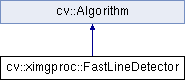OpenCV  3.4.10 Open Source Computer Vision
cv::ximgproc::FastLineDetector Class Referenceabstract

Class implementing the FLD (Fast Line Detector) algorithm described in  . More...

#include <opencv2/ximgproc/fast_line_detector.hpp>

Inheritance diagram for cv::ximgproc::FastLineDetector:## Public Member Functions

virtual ~FastLineDetector ()

virtual void detect (InputArray _image, OutputArray _lines)=0
Finds lines in the input image. This is the output of the default parameters of the algorithm on the above shown image. More...

virtual void drawSegments (InputOutputArray _image, InputArray lines, bool draw_arrow=false)=0
Draws the line segments on a given image. More...Public Member Functions inherited from cv::Algorithm
Algorithm ()

virtual ~Algorithm ()

virtual void clear ()
Clears the algorithm state. More...

virtual bool empty () const
Returns true if the Algorithm is empty (e.g. in the very beginning or after unsuccessful read. More...

virtual String getDefaultName () const

virtual void read (const FileNode &fn)
Reads algorithm parameters from a file storage. More...

virtual void save (const String &filename) const

virtual void write (FileStorage &fs) const
Stores algorithm parameters in a file storage. More...

void write (const Ptr< FileStorage > &fs, const String &name=String()) const
simplified API for language bindings This is an overloaded member function, provided for convenience. It differs from the above function only in what argument(s) it accepts. More...Static Public Member Functions inherited from cv::Algorithm
template<typename _Tp >
static Ptr< _Tp > load (const String &filename, const String &objname=String())
Loads algorithm from the file. More...

template<typename _Tp >
static Ptr< _Tp > loadFromString (const String &strModel, const String &objname=String())
Loads algorithm from a String. More...

template<typename _Tp >
static Ptr< _Tp > read (const FileNode &fn)
Reads algorithm from the file node. More...Protected Member Functions inherited from cv::Algorithm
void writeFormat (FileStorage &fs) const

## Detailed Description

Class implementing the FLD (Fast Line Detector) algorithm described in  .

#include <iostream>
using namespace std;
using namespace cv;
using namespace cv::ximgproc;
int main(int argc, char** argv)
{
std::string in;
cv::CommandLineParser parser(argc, argv, "{@input|../samples/data/corridor.jpg|input image}{help h||show help message}");
if (parser.has("help"))
{
parser.printMessage();
return 0;
}
in = parser.get<string>("@input");
if( image.empty() )
{
return -1;
}
// Create FLD detector
// Param Default value Description
// length_threshold 10 - Segments shorter than this will be discarded
// distance_threshold 1.41421356 - A point placed from a hypothesis line
// segment farther than this will be
// regarded as an outlier
// canny_th1 50 - First threshold for
// hysteresis procedure in Canny()
// canny_th2 50 - Second threshold for
// hysteresis procedure in Canny()
// canny_aperture_size 3 - Aperturesize for the sobel
// operator in Canny()
// do_merge false - If true, incremental merging of segments
// will be perfomred
int length_threshold = 10;
float distance_threshold = 1.41421356f;
double canny_th1 = 50.0;
double canny_th2 = 50.0;
int canny_aperture_size = 3;
bool do_merge = false;
distance_threshold, canny_th1, canny_th2, canny_aperture_size,
do_merge);
vector<Vec4f> lines_fld;
// Because of some CPU's power strategy, it seems that the first running of
// an algorithm takes much longer. So here we run the algorithm 10 times
// to see the algorithm's processing time with sufficiently warmed-up
// CPU performance.
for(int run_count = 0; run_count < 10; run_count++) {
double freq = getTickFrequency();
lines_fld.clear();
int64 start = getTickCount();
// Detect the lines with FLD
fld->detect(image, lines_fld);
double duration_ms = double(getTickCount() - start) * 1000 / freq;
std::cout << "Elapsed time for FLD " << duration_ms << " ms." << std::endl;
}
// Show found lines with FLD
Mat line_image_fld(image);
fld->drawSegments(line_image_fld, lines_fld);
imshow("FLD result", line_image_fld);
return 0;
}

## ◆ ~FastLineDetector()

 virtual cv::ximgproc::FastLineDetector::~FastLineDetector ( )
inlinevirtual

## ◆ detect()

 virtual void cv::ximgproc::FastLineDetector::detect ( InputArray _image, OutputArray _lines )
pure virtual
Python:
_lines=cv.ximgproc_FastLineDetector.detect(_image[, _lines])

Finds lines in the input image. This is the output of the default parameters of the algorithm on the above shown image.image
Parameters
 _image A grayscale (CV_8UC1) input image. If only a roi needs to be selected, use: fld_ptr-\>detect(image(roi), lines, ...); lines += Scalar(roi.x, roi.y, roi.x, roi.y); _lines A vector of Vec4f elements specifying the beginning and ending point of a line. Where Vec4f is (x1, y1, x2, y2), point 1 is the start, point 2 - end. Returned lines are directed so that the brighter side is on their left.
Examples:
fld_lines.cpp.

## ◆ drawSegments()

 virtual void cv::ximgproc::FastLineDetector::drawSegments ( InputOutputArray _image, InputArray lines, bool draw_arrow = false )
pure virtual
Python:
_image=cv.ximgproc_FastLineDetector.drawSegments(_image, lines[, draw_arrow])

Draws the line segments on a given image.

Parameters
 _image The image, where the lines will be drawn. Should be bigger or equal to the image, where the lines were found. lines A vector of the lines that needed to be drawn. draw_arrow If true, arrow heads will be drawn.
Examples:
fld_lines.cpp.

The documentation for this class was generated from the following file: